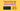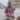# How to Solve the Infinite Loop of React.useEffect()

Posted January 19, 2021Start discussion

`useEffect()` React hook manages the side-effects like fetching over the network, manipulating DOM directly, starting and ending timers.

While the `useEffect()` is, alongside with `useState()` (the one that manages state), is one of the most used hooks, it requires some time to familiarize and use correctly.

A pitfall you might experience when working with `useEffect()` is the infinite loop of component renderings. In this post, I’ll describe the common scenarios that generate infinite loops and how to avoid them.

If you aren’t familiar with `useEffect()`, I recommend reading my post A Simple Explanation of React.useEffect() before continuing. Having good fundamental knowledge of a non-trivial subject helps bypass the rookie mistakes.

## 1. The infinite loop and side-effect updating state

A functional component contains an input element. Your job is to count and display how many times the input has changed.

A possible implementation of `<CountInputChanges>` component looks as follows:

``````import { useEffect, useState } from 'react';

function CountInputChanges() {
const [value, setValue] = useState('');
const [count, setCount] = useState(-1);

useEffect(() => setCount(count + 1));
const onChange = ({ target }) => setValue(target.value);

return (
<div>
<input type="text" value={value} onChange={onChange} />
<div>Number of changes: {count}</div>
</div>
)
}``````

`<input type="text" value={value} onChange={onChange} />` is a controlled component. `value` state variable holds the input value, and the `onChange` event handler updates the `value` state when user types into the input.

I took the decision to update the `count` variable using `useEffect()` hook. Every time the component re-renders due to user typing into the input, the `useEffect(() => setCount(count + 1))` updates the counter.

Because `useEffect(() => setCount(count + 1))` is used without dependencies argument, `() => setCount(count + 1)` callback is executed after every rendering of the component.

Do you expect any problems with this component? Take a try and open the demo.

The demo shows that `count` state variable increases uncontrollably, even if you haven’t typed anything into the input. That’s an infinite loop.

The problem lays in the way `useEffect()` is used:

``useEffect(() => setCount(count + 1));``

it generates an infinite loop of component re-renderings.

After initial rendering, `useEffect()` executes the side-effect callback that updates the state. The state update triggers re-rendering. After re-rendering `useEffect()` executes the side-effect callback and again updates the state, which triggers again a re-rendering. …and so on indefinitely.

### 1.1 Fixing dependencies

The infinite loop is fixed by correct management of the `useEffect(callback, dependencies)` dependencies argument.

Because you want the `count` to increment when `value` changes, you can simply add `value` as a dependency of the side-effect:

``````import { useEffect, useState } from 'react';

function CountInputChanges() {
const [value, setValue] = useState('');
const [count, setCount] = useState(-1);

useEffect(() => setCount(count + 1), [value]);
const onChange = ({ target }) => setValue(target.value);

return (
<div>
<input type="text" value={value} onChange={onChange} />
<div>Number of changes: {count}</div>
</div>
);
}``````

Adding `[value]` as a dependency of `useEffect(..., [value])`, the `count` state variable is updated only when `[value]` is changed. Doing so solves the infinite loop.

Open the fixed demo. Now, as soon as you type into the input field, the `count` state correctly display the number of input value changes.

### 1.2 Using a reference

An alternative to the above solution is to use a reference (created by useRef() hook) to store the number of changes of the input.

The idea is that updating a reference doesn’t trigger re-rendering of the component.

Here’s a possible implementation:

``````import { useState, useRef } from 'react';

function CountInputChanges() {
const [value, setValue] = useState('');
const countRef = useRef(0);

const onChange = ({ target }) => {
setValue(target.value);
countRef.current++;  };

return (
<div>
<input type="text" value={value} onChange={onChange} />
<div>Number of changes: {countRef.current}</div>
</div>
);
}``````

Inside the event handler `onChange` the `countRef.current++` is executed each time the `value` state changes. The reference change doesn’t trigger re-rendering.

Check out the demo. Now, as soon as you type into the input field, the `countRef` reference is updated without triggering a re-rendering — efficiently solving the infinite loop problem.

## 2. The infinite loop and new objects references

Even if you set up correctly the `useEffect()` dependencies, still, you have to be careful when using objects as dependencies.

For example, the following component `CountSecrets` watches the words the user types into the input, and as soon as the user types the special word `'secret'`, a counter of secrets is increased and displayed.

Here’s a possible implementation of the component:

``````import { useEffect, useState } from "react";

function CountSecrets() {
const [secret, setSecret] = useState({ value: "", countSecrets: 0 });

useEffect(() => {
if (secret.value === 'secret') {
setSecret(s => ({...s, countSecrets: s.countSecrets + 1}));    }
}, [secret]);
const onChange = ({ target }) => {
setSecret(s => ({ ...s, value: target.value }));
};

return (
<div>
<input type="text" value={secret.value} onChange={onChange} />
<div>Number of secrets: {secret.countSecrets}</div>
</div>
);
}``````

Open the demo and type some words, one of which to be `'secret'`. As soon as you type the word `'secret'`, the `secret.countSecrets` state variable starts to grow uncontrollably.

That’s an infinite loop problem.

Why does it happen?

The `secret` object is used as a dependency of `useEffect(..., [secret])`. Inside the side-effect callback, as soon as the input value equals `'secret'`, the state updater function is called:

``setSecret(s => ({...s, countSecrets: s.countSecrets + 1}));``

which increments the secrets counter `countSecrets`, but also creates a new object.

`secret` now is a new object and the dependency has changed. So `useEffect(..., [secret])` invokes again the side-effect that updates the state and a new `secret` object is created again, and so on.

2 objects in JavaScript are equal only if they reference exactly the same object.

### 2.1 Avoid objects as dependencies

The best way to solve the problem of an infinite loop created by circular new objects creation is… to avoid using references to objects in the dependencies argument of `useEffect()`:

``````let count = 0;

useEffect(() => {
// some logic
}, [count]); // Good!``````
``````let myObject = {
prop: 'Value'
};

useEffect(() => {
// some logic
}, [myObject]); // Not good!
useEffect(() => {
// some logic
}, [myObject.prop]); // Good!``````

Fixing the infinite loop of `<CountSecrets>` component requires changing the dependency from `useEffect(..., [secret])` to `useEffect(..., [secret.value])`.

Calling the side-effect callback when solely `secret.value` changes is enough. Here’s the fixed version of the component:

``````import { useEffect, useState } from "react";

function CountSecrets() {
const [secret, setSecret] = useState({ value: "", countSecrets: 0 });

useEffect(() => {
if (secret.value === 'secret') {
setSecret(s => ({...s, countSecrets: s.countSecrets + 1}));
}
}, [secret.value]);
const onChange = ({ target }) => {
setSecret(s => ({ ...s, value: target.value }));
};

return (
<div>
<input type="text" value={secret.value} onChange={onChange} />
<div>Number of secrets: {secret.countSecrets}</div>
</div>
);
}``````

Open the fixed demo. Type some words into the input… and as soon as you enter the special word `'secret'` the secrets counter increments. No infinite loop is created.

## 3. Summary

`useEffect(callback, deps)` is the hook that executes `callback` (the side-effect) after the component rendering. If you aren’t careful with what the side-effect does, you might trigger an infinite loop of component renderings.

A common case that generates an infinite loop is updating state in the side-effect without having any dependency argument at all:

``````useEffect(() => {
// Infinite loop!
setState(count + 1);
});``````

An efficient way to avoid the infinite loop is to properly manage the hook dependencies — control when exactly the side-effect should run.

``````useEffect(() => {
// No infinite loop
setState(count + 1);
}, [whenToUpdateValue]);``````

Alternatively, you can also use a reference. Updating a reference doesn’t trigger a re-rendering:

``countRef.current++;``

Another common recipe of an infinite loop is using an object as a dependency of `useEffect()`, and inside the side-effect updating that object (effectively creating a new object):

``````useEffect(() => {
// Infinite loop!
setObject({
...object,
prop: 'newValue'
})
}, [object]);``````

Avoid using objects as dependencies, but stick to use a specific property only (the end result should be a primitive value):

``````useEffect(() => {
// No infinite loop
setObject({
...object,
prop: 'newValue'
})
}, [object.whenToUpdateProp]);``````

What are other common mistakes when using React hooks? In one of my previous posts I talked about 5 Mistakes to Avoid When Using React Hooks.

What other infinite loop pitfalls when using `useEffect()` do you know?

## Quality posts into your inbox

I regularly publish posts containing:

• Important JavaScript concepts explained in simple words
• Overview of new JavaScript features
• How to use TypeScript and typing
• Software design and good coding practices